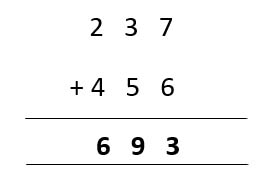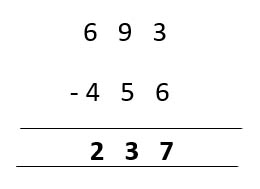# 2nd Grade Addition and Subtraction: Key Terms and Facts Every Student Should Know

• Hi everyone! Welcome to another enriching resource about 2nd-grade math. In this article, we will learn about 2nd Grade addition and subtraction, two of the most important skills that early math learners need to master. We will also review some key terms and facts that every student should know to accurately understand and solve math problems.

And, of course, we will have some fun and easy practice exercises to test your knowledge and skills. Are you ready? Let's get started!

## Introduction: Why 2nd Grade math matters

Before understanding what addition and subtraction mean, we must determine why 2nd Grade matters.

First, math is everywhere in our lives. We use it to count, measure, compare, and more. Also, it helps us make sense of the world and solve problems. That's why it's important to learn math well and enjoy it.

In 2nd grade, you will learn more about numbers and how to use them differently. You will also learn how to add and subtract numbers up to 1000. These are essential skills that you will use throughout your life.

• ### Addition: What it means and how to do it

The addition is one of the basic operations in math. It means combining two or more numbers to get a bigger number. For example, if you have 3 apples and 4 oranges, add them together to get 7 fruits.

The symbol for addition is +. To add two numbers, you must line them up according to their place values (ones, tens, hundreds, etc.) and then add each pair of digits from right to left. If a pair of digits sum is 10 or more, you must carry the extra digit to the next place value.

For example:In this example, we start by adding the ones: 7 + 6 = 13. Since this is more than 10, we write 3 in the ones place and carry over 1 to the tens place.

Then we add the tens: 1 + 3 + 5 = 9. We write 9 in the tens place.

Finally, we add the hundreds: 2 + 4 = 6. We write 6 in the hundreds place.

• ### Subtraction: What it means and how to do it

Subtraction is another basic operation in math. It means taking away one number from another number to get a smaller number. For example, if you have 7 candies and eat 4 of them, you can subtract them to find out how many candies you have left.

The symbol for subtraction is -. To subtract two numbers, you need to line them up according to their place values (ones, tens, hundreds, etc.) and then subtract each pair of digits from right to left. If the digit on the top is smaller than the digit on the bottom, you must borrow a digit from the next place value.In this example, we start by subtracting the ones: 3 - 6 = -3. Since this is negative, we borrow a digit from the tens place and make it 13 - 6 = 7. We write 7 in the ones place and reduce the tens place by one.

Then we subtract the tens: 8 - 5 = 3. We write 3 in the tens place.

Finally, we subtract the hundreds: 5 - 4 = 1. We write 1 in the hundreds place.

• ### Key Terms: Words you need to know for 2nd-grade math

There are some words that you need to know for 2nd-grade math. These words help learners understand what the math problems ask them to do and how to explain their answers.

Here are some of the most common words:

• Addend: A number that is added to another number. For example, in 3 + 4 = 7, both 3 and 4 are addends.
• Sum: The answer to an addition problem. For example, in the addition sentence, 3 + 4 = 7; the number 7 is the sum.
• Minuend: A number that is subtracted from another number. For example, in the subtraction sentence, 7 - 4 = 3, the number 7 is the minuend.
• Subtrahend: A number that is subtracted from another number. For example, in 7 - 4 = 3, the number 4 is the subtrahend.
• Difference: The answer to a subtraction problem. For example, in the subtraction sentence, 7 - 4 = 3, the number 3 is the difference.
• Place value: The value of a digit depending on its position in a number. For example, in 237, the 2 has a place value of hundreds, the 3 has a place value of tens, and the number 7 has a place value of ones.
• Regrouping: The process of rearranging digits in a number to make adding or subtracting easier. For example, in 237 + 456, we can regroup 237 as 200 + 30 + 7 and 456 as 400 + 50 + 6. This makes it easier to add each pair of digits.
• ### Facts: Basic rules and strategies for addition and subtraction

There are some facts or basic rules and strategies that you need to know for addition and subtraction. These facts help you do math faster and more accurately.

Here are some of the most important facts:

• The commutative property means that you can change the order of the addends without changing the answer.

For example, 3 + 4 = 4 + 3.

• The associative property means that you can change the grouping of the addends without changing the answer. For example, (3 + 4) + 5 = 3 + (4 + 5).
• The identity property means that adding zero or subtracting zero does not change the number. For example, 3 + 0 = 3 and 3 - 0 = 3.
• Inverse property means that adding the opposite or subtracting the same number gives zero. For example, 3 + (-3) = 0 and   3 - 3 = 0.
• Addition and subtraction facts: These are the basic combinations of numbers that you need to memorize. For example, you need to know that 2 + 2 = 4, 5 - 3 = 2, etc.

Knowing these facts will help you do math faster, mentally, and more confidently.

### Practice: Fun and easy exercises to master 2nd Grade Math

Now that you have learned some key terms and facts about addition and subtraction, it's time to practice what you have learned. Here are some fun and easy exercises that you can do to master 2nd-grade math:

• Fill in the blanks: Use the commutative property to fill in the missing numbers. For example,

5 + 6 = 6 + 5.

10 + 7 = __ + __

45 + 12 = __ + __

246 + 128= __ + __

• Solve the problems: Use the associative property to solve the problems. For example,

(2 + 3) + 4 = 2 + (3 + 4)

(23 + 16) + 9 = ________

7 + (18 + 30) = ________

9 + 12 + 10 = ________

• Find the missing numbers: Use the identity property to find the missing numbers. For example, 7 + 0 = 7.

43 + 0 = _

12 – 0 = _

60 - 0 =  _

• Find the opposites: Use the inverse property to find the opposites of the numbers. For example, the opposite of -5 is 5.

The opposite of -8 is _

The opposite of -10 is _

The opposite of -12 is _

• Write the facts: Write down all the addition and subtraction facts for each number from 0 to 10. For example, for 0: we know that 0 + 0 = 0 and 0 - 0 = 0

&helpp;

...

Subtraction facts:

...

...

Thank you for sharing the links of MathSkills4Kids.com with your loved ones. Your choice is greatly appreciated.

### Conclusion: How to apply what you learned in real life

Congratulations! You and your kids have learned a lot about addition and subtraction in this article. The kids have learned what addition and subtraction mean, how to do them, some key terms and facts, and some practice exercises. But how can they apply what they've learned in real life?

Here are some examples:

• You can use addition and subtraction to count money, measure length, weight, or time, compare quantities, etc.
• You can use addition and subtraction to solve word problems that involve adding or subtracting numbers or objects.
• You can use addition and subtraction to check your answers by doing the opposite operation. For example, if you added two numbers and got an answer, you can subtract one of them from the answer to get back the other number.

I hope you find these resources helpful in reinforcing your 2nd graders' addition and subtraction properties skills. Remember that practice makes perfect, but it doesn't have to be boring! With these resources, your kids will have fun while learning math.

Happy learning!

•